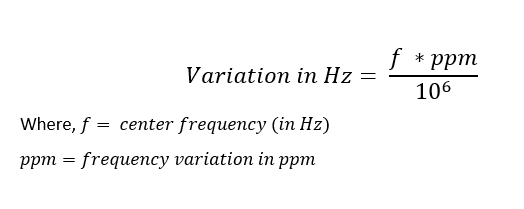Frequency stability represents the variation of output frequency of a crystal oscillator due to external conditions like temperature variation, voltage variation, output load variation, and frequency aging. Frequency stability is typically expressed in parts per million (ppm) or parts per billion (ppb) which can be represented in the form of frequency (usually in Hz).

Parts per Million (ppm): 1 ppm means 1/106 part of a nominal frequency.For example – If a quartz oscillator has an output frequency of 1 MHz (1000000 Hz) and it has a Frequency Stability of 5 ppm, it will vary in frequency by 5 Hz.

Stability tolerance can also be expressed as a percentage of frequency deviation rather than as parts per million (ppm).

0.01 % = 100 ppm

0.005% = 50 ppm

0.001 % = 10 ppm

0.0001% = 1 ppm

In conclusion, Frequency Stability is inclusive of calibration @ 25°C, operating temperature range, supply voltage change, load change, ageing, shock and vibration.